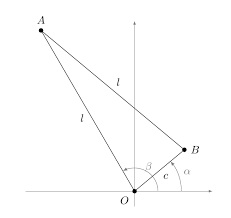# Right Angle's Vertex Is On The Line!

Algebra Level 5Suppose we have two points $A(5, 5)$ and $B(3, 2)$. Point C is placed on the line $y = 3x+4$ such that $\triangle ABC$ is a right triangle.

If $C$ has coordinates $(x, y)$ and the sum of all possible values of $(x, y)$ is $P$, then find $11P$.

×